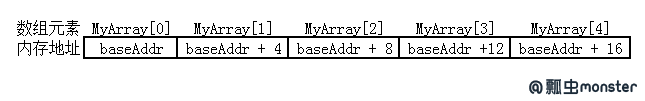## 我所理解的Delphi中的数组类型

var
MyArray: array[0..4] of Integer;        { 声明一个数组包括5个整数数值}
begin
MyArray := -200;                     { 通过操作符[]就可以访问每个数组元素}
MyArray := -100;
MyArray := 0;
MyArray := 100;
MyArray := 200;
MyArray := MyArray + MyArray;  { MyArray为-100}
end;1、多维数组

const
CArray: array[0..4] of Integer = (-20, -100, 0, 100, 200);
{ 数组常量的在声明的同时也要进行赋初值}
var
MyArray: array[0..2, 0..4] of Integer; { 两维数组的声明}
UArray: array[10..20] of Integer;      { 声明了一个下界10到上界20的11个元素的数组}
X: Integer;
begin                                    { 两种方法可以访问两维数组}
X := MyArray + MyArray;    { 1、[X][Y]访问}
X := MyArray[1, 1] + MyArray[2, 1];    { 2、[X, Y]访问}

{ 下面的访问超出了数组范围，
将会提示“Constant expression violates subrange bounds”错误}
X := MyArray[3, 1] + MyArray[0, 5];    {注意这两个元素超出数组声明的范围}
end;

2、上界与下界

var
X, I, Lower, Upper: Integer;
MyArray: array[10..20] of Integer;
begin
{ 这里假使已经将MyArray数组进行了赋初值}
Lower := Low(MyArray); { Lower的值为 10}
Upper := High(MyArray);{ Upper的值为 20}
X := 0；
for I := Lower to Upper do
begin
X := X + MyArray[I]; { X将累加数组元素值的和}
end;
end;

var
Lower1, Upper1: Integer;{ 用来存储一维的上下界}
Lower2, Upper2: Integer;{ 用来存储二维的上下界}
MyArray: array[10..20, 1..2] of Integer;
begin
{ 这里假使已经将MyArray数组进行了赋初值}
Lower1 := Low(MyArray); { Lower的值为 10}
Upper1 := High(MyArray);{ Upper的值为 20}
ShowMessage('第一维的下界为 ' + IntToStr(Lower1) +
'，上界为 ' + IntToStr(Upper1));
Lower2 := Low(MyArray[Lower1]); {获取MyArray下界}
Upper2 := High(MyArray[Lower1]);{获取MyArray上界}
ShowMessage('第二维的下界为 ' + IntToStr(Lower2) +
'，上界为 ' + IntToStr(Upper2));
end;

3、动态数组(dynamic array)

var
SA: array of string;                 { 一维动态数组}
begin
{ 使用SetLength进行动态数组的空间分配，已有元素可以得到保留}
SetLength(SA, 3);
SA := 'Hello World';
{ 重新分配了动态数组大小为2个元素}
SetLength(SA, 2);
ShowMessage(SA);  {显示为'Hello World',说明已有元素得到保留}
end;

var
SA: array of array of string;   { 二维动态数组}
begin
{ 使用SetLength进行动态数组的空间分配，已有元素可以得到保留}
SetLength(SA, 20, 20);
SA := 'Hello World';
{ 重新分配了动态数组大小为2个元素}
SetLength(SA, 10, 10);
ShowMessage(SA);  {显示为'Hello World',说明已有元素得到保留}
end;

var
SA: array of string;
begin
SetLength(SA, 20);
SA := 'Hello World';
SA := nil; { 直接把nil赋值给SA就可以了}
end;

var
A1, A2: array of Integer;
begin
SetLength(A1, 4);
A2 := A1;
A1 := 1;
A2 := 26;
ShowMessage(IntToStr(A1)); { 显示结果为 26}
{
为什么显示26呢？因为A2 := A1这条赋值语句，并没有创建新的数组，仅仅只是将
A1数组的引用赋值给了A2，也就是说A2只是A1的一个别名而已，指向的都是相同东西，
因此对A2所做的任何操作都会影响到A1，如果想要完全复制创建一个新的A2数组需要
用到Copy函数，如下：
}

A2 := Copy(A1); { A1, A2现在为两个独立的数组}
A2 := 10;
A1 := 26;
ShowMessage(IntToStr(A2));  { 现在A2的值为10，说明完全的独立了}
A2 := nil; {释放掉A2}

A2 := Copy(A1, 1, 2); { 从元素1开始，复制2个元素到A2}
end;

posted on 2011-12-15 13:09  pchmonster  阅读(10525)  评论(6编辑  收藏  举报